# ISEE Middle Level Math : Parallelograms

## Example Questions

### Example Question #1 : How To Find The Perimeter Of A Parallelogram

36 in

26 in

13 in

18 in

26 in

Explanation:

To find the perimeter of a parallelogram, add the lengths of the sides. Opposite sides of a parallelogram are equivalent.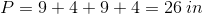### Example Question #2 : How To Find The Perimeter Of A Parallelogram

If the perimter of a parallelogram isand one of the sides is, what is the other side length?Explanation:

The perimeter of a parallelogram is found by adding up all four sides.

Since there are two pairs of side with equal lengths, two sides must have a length of.

So the perimeter would be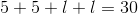or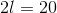.

To find the value of the other side length, you would divide the remaining perimeter not include in the other sides by.

So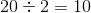.

That means the other side length would be.

### Example Question #3 : How To Find The Perimeter Of A Parallelogram

If a parallelogram has side lengths ofand, what is the perimeter?Explanation:

The perimeter of a parallelogram is two times the two side lengths and add them together.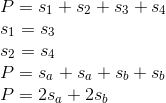Therefore, this particular problem becomes as follows.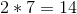and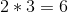so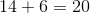.

### Example Question #1 : How To Find The Perimeter Of A Parallelogram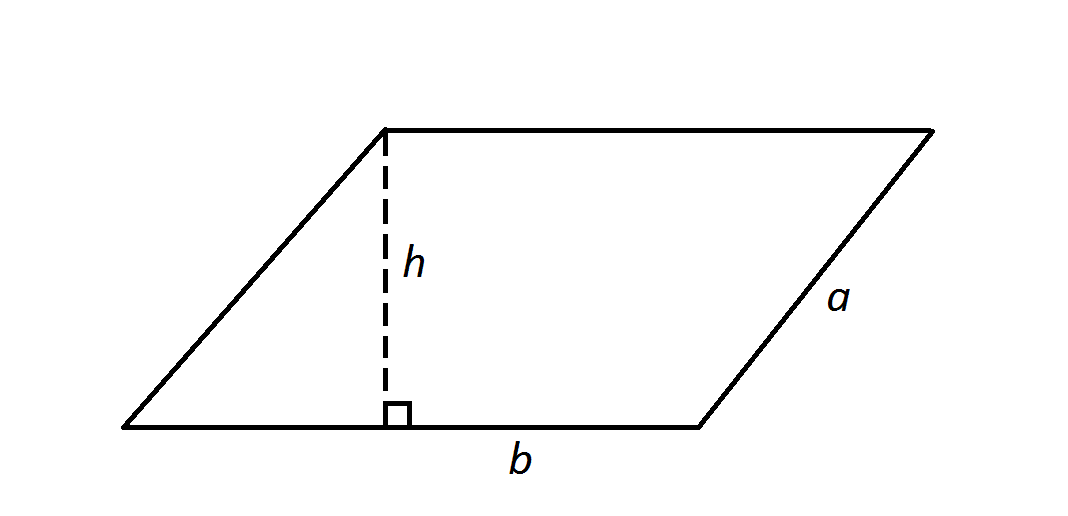Note: Figure NOT drawn to scale.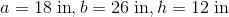, where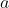and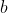represent side lengths of the parallelogram and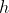represents the height.

Find the perimeter of the parallelogram in the diagram.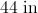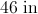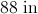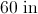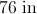Explanation:

The perimeter of the parallelogram is the sum of the four side lengths - here, that formula becomes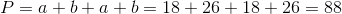.

Note that the heightis irrelevant to the answer.

### Example Question #1 : How To Find The Area Of A Parallelogram

Find the area of the following parallelogram: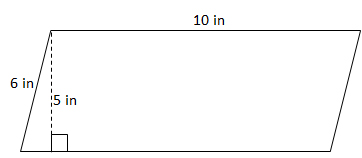Note: The formula for the area of a parallelogram is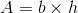.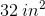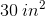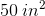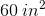Explanation:

The base of the parallelogram is 10, while the height is 5.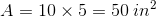### Example Question #2 : How To Find The Area Of A Parallelogram

Find the area: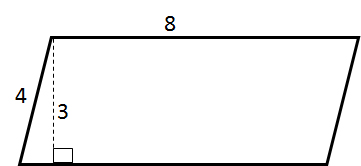Explanation:

The area of a parallelogram can be determined using the following equation: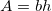Therefore,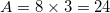### Example Question #3 : How To Find The Area Of A Parallelogram

You can solve the area of a parallelogram when you know the lengths of each of the sides. True or False?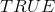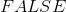Explanation:

The area of a parallelogram is found by computing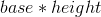.  In this situation, you would have the base which is the bottom side but you would not have the height measurement.  Since you would not be able to solve for the area with just the side lengths, the statement is.

### Example Question #4 : How To Find The Area Of A Parallelogram

If a parallelogram has side lengths ofand, what is the area?Cannot be determined.Cannot be determined.

Explanation:

To find the area of a parallelogram, you use the formula,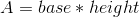.

Since the height in this problem is not known, you cannot solve for area.

### Example Question #5 : How To Find The Area Of A Parallelogram

Find the area of a parallelogram with a base of 6 inches and a height of 9 inches.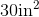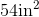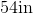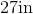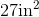Explanation:

To find the area of a parallelogram, we will use the following formula: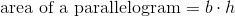where is the base and h is the height of the parallelogram.

Now, we know the base has a length of 6 inches. We also know it has a height of 9 inches.  Knowing this, we can substitute into the formula.  We get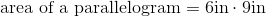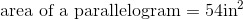### Example Question #6 : How To Find The Area Of A Parallelogram

Find the area of the parallelogram with a base length of 6 and a height of 15.Explanation:

Write the area formula of a parallelogram.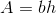Substitute the dimensions into the formula.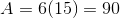The answer is: